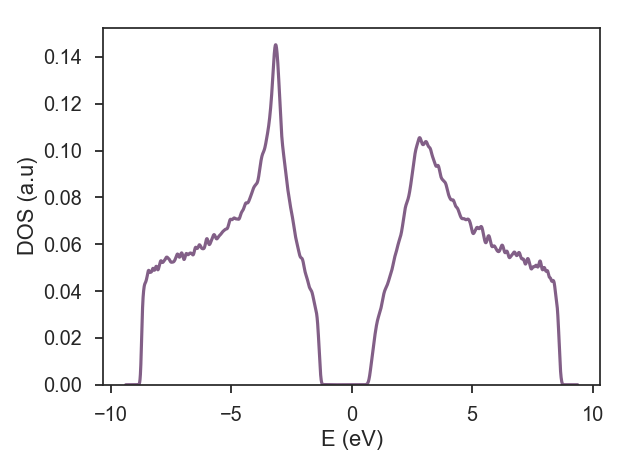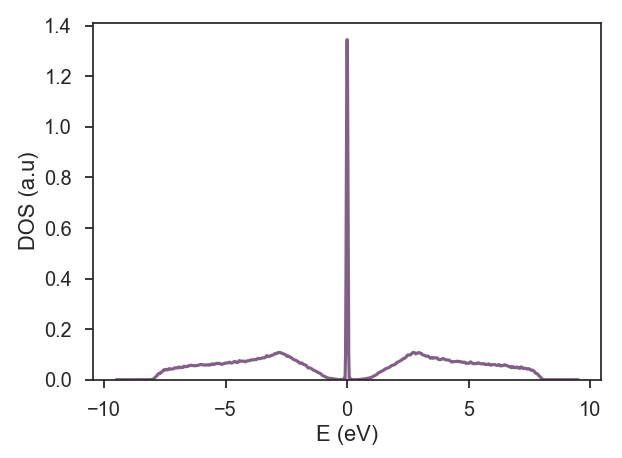The purpose of this section is to provide a simple overview of the different types of disorder that can be added to KITE tight-binding calculations. The general character of our disorder implementation is one of the main features of KITE. To achieve this generality, the implementation follows a basic structure: the user specifies the disorder pattern to be included (that can be constricted to one unit cell or can connect neighboring unit cells) and the disorder pattern is reproduced randomly inside the sample, according to a defined concentration and statistical distribution..

After defining a lattice with the procedure explained in Getting Started), we can add disorder to our system. Usually, disorder can be modeled either as a modification of onsite potentials appearing on the lattice sites or as a combination of onsite potential and bond disorder. Hence, KITE allows the user to select between the two types of disorder by choosing between predefined classes in the python interface. The interface provides two different classes of disorder: * Disorder – onsite disorder with three possible statistical distributions * StructuralDisorder – generic structural disorder, the combination of onsite potential and bond disorder.

# Onsite disorder

`Disorder` adds randomly generated onsite terms at the sites of a desired sublattice based on a certain statistical distribution:

• Gaussian;
• Uniform;
• Deterministic.

Beside the type of statistical distribution, we can select a sublattice type in which the disorder will appear, the mean value and the standard deviation of the selected distribution. To include onsite disorder following a given statistical distribution, we build the `lattice` and use the following procedure:

``````disorder = kite.Disorder(lattice) # define an object based on the lattice
disorder.add_disorder('A', 'Gaussian', 0.1, 0.1) # add Gaussian distributed disorder at all sites of a selected sublattice
``````

In a single object it is possible to select multiple sublattices, each of one with different disorder distributions following the rule `disorder.add_disorder('sublattice', 'type', mean, std)` :

``````disorder.add_disorder('A', 'Gaussian', 0.1, 0.1)
``````

In the case of deterministic disorder, the standard deviation is not set. These quantities are in the same units as the ones specified in the rest of the configuration file.

After defining the desired disorder, it can be added to the configuration file as an additional parameter in the `config_system` function:

``````kite.config_system(..., disorder=disorder)
``````

A complete example that calculates the density of states of graphene with different on-site disorder distributions for each sublattice can be seen here:

with the resulting density of states:# Structural disorder

`StructuralDisorder` class adds the possibility of selecting between two different structural disorder types; vacancy, randomly distributed with a certain concentration in sites of a selected sublattice, and a more generic structural disorder which is a combination of onsite terms and bond disorder (also distributed with a certain concentration).

## Vacancy disorder

The vacant site distribution can be selected from a single sublattice with a concentration defined in a parent object:

``````struc_disorder = kite.StructuralDisorder(lattice, concentration=0.2)

``````

IMPORTANT: to distribute the vacancies in both sublattices, one needs to add the vacancies on each sublattice as a separate object of the class `StructuralDisorder` (unless you one precisely the same pattern of disorder in both sublatices)

``````struc_disorder_A = kite.StructuralDisorder(lattice, concentration=0.1)
struc_disorder_B = kite.StructuralDisorder(lattice, concentration=0.1)
disorder_structural = [struc_disorder_A, struc_disorder_B]
``````

## Structural disorder

Before discussing this class of disorder, it is important to mention that in the pre-release version, it is no possible to perform the automatic scale of the spectra for hopping disorder. In this case, it is necessary to add an extra parameter to the configuration class:

``````configuration = kite.Configuration(divisions=[nx, ny], length=[lx, ly], boundaries=[True, True],is_complex=False, precision=1,spectrum_range=[-10, 10])
``````

The following example shows a definition of our most general type of disorder, which includes both onsite disorder terms and bond modifications. This type of disorder can be added as an object of the class `StructuralDisorder`. The procedure for adding the structural disorder is the same of adding a hopping term to the Pybinding lattice object, with a single difference that the bond disorder is not bounded to the hopping term starting from the [0, 0] unit cell, which is the case of the hopping term in pybinding.

For the sake of clarity, let us first define sublattices that will compose the disorder. In this case we are not restricted to a single unit cell:

``````node0 = [[+0, +0], 'A'] # define a node in a unit cell [i, j] selecting a single sublattice
node1 = [[+0, +0], 'B']
node2 = [[+1, +0], 'A']
node3 = [[+0, +1], 'B']
node4 = [[+0, +1], 'A']
node5 = [[-1, +1], 'B']
``````

After the definition of a parent `StructuralDisorder` object, we can select the desired pattern:

``````
struc_disorder = kite.StructuralDisorder(lattice, concentration=0.2) # define an object based on the lattice with a certain concentration

# add bond disorder in the form [from unit cell], 'sublattice_from', [to_unit_cell], 'sublattice_to', value:
(*node0, *node1, 0.5),
(*node1, *node2, 0.1),
(*node2, *node3, 0.5),
(*node3, *node4, 0.3),
(*node4, *node5, 0.4),
(*node5, *node0, 0.8),
# in this way we can add onsite disorder in the form [unit cell], 'sublattice', value
([+0, +0], 'B', 0.1)
)
# It is possible to add multiple different disorder types which should be forwarded to the config_system function
# as a list.
another_struc_disorder = kite.StructuralDisorder(lat, concentration=0.6)
(*node0, *node1, 0.05),
(*node4, *node5, 0.4),
(*node5, *node0, 0.02),
([+0, +0], 'A', 0.3)
)
``````

Before exporting the settings to the hdf file, it is possible to define multiple disorder realizations which will be superimposed to the clean system.

The following script has a a minimal example of how to configure the structural disorder

with the resulting density of states